# Volumes of solids of revolution - Shell method - Integration Applications

## Shell Method

Shell method (also known as the method of cylindrical shells) is another method that is used in finding the volume a solid. To be more precise, shell method is used when the rotation of the function creates cylinder-like shells as the cross section. Once you get the area of the cylindrical shells, then integrating it will give us the volume of the solid. It is similar to the disk method and washer method because it involves solids of revolution, but the process in using shell's method is slightly different. The Shell Method formula is one of: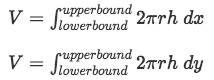Formula 1: Shell Method Formulas

where r is the radius and h is the height. The first one is used for shell method around y axis, and the second one is shell method around x axis. Shell method can even be used for rotations around specific x and y values.

The interesting thing is that if we were to integrate the area of the cylindrical shell, then we actually get the volume of cylinder. Look at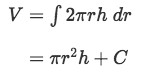Formula 2: Volume of a cylinder

Ignoring the constant of integration, we see πr2 h is the cylinder volume formula.

Now here is a question you are probably asking. Why do we introduce another method? This is because there some cases where the disc method (or washer method) actually fails, and cannot calculate the volume of a solid. Let me give you an example.

## Shell Method With Y Axis Rotation

Question 1: Find the volume of the solid obtained by rotating the region bounded by the x-axis and the following curve about the y-axis.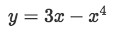Equation 1: Shell Method about y axis pt.1

Since we are dealing with two functions (x-axis and the curve), we are going to use the washer method here. Recall that the washer method formula for y-axis rotation is: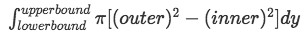Equation 1: Shell Method about y axis pt.2

Where outer is the outer radius of the circle, and inner is the inner radius of the circle.

Notice that the curve looks like this:Graph 1: Shell Method about y axis pt.3

Rotating it around the y-axis will show us the inner radius and outer radiusGraph 2: Shell Method about y axis pt.4

However, notice that both the inner radius and outer radius occur on the same function. So if we were to use the washer-method formula, then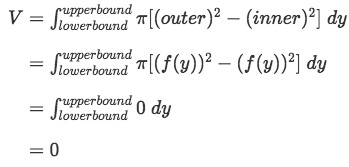Equation 1: Shell Method about y axis pt.5

Obviously the volume is not 0, so the washer method fails here. Now let's actually do the question with shell method.

• Since the region is bounded by the x-axis and the curve, then we would like to find the intersection to get the bounds. Notice that the x-axis is the equation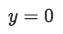Equation 1: Shell Method about y axis pt.6
• So setting y=0 and the curve equal gives: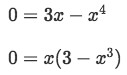• We see that the solutions for x are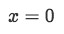Equation 1: Shell Method about y axis pt.8

And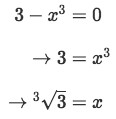Equation 1: Shell Method about y axis pt.9
• So our lower bound will be a 0 and the upper bound will be the cube root of 3. Now drawing the curves and plotting those bounds gives us the following graph:Graph 3: Shell Method about y axis pt.10
• Now what were going to do is rotate this around the y-axis, and show you how to imagine the cylindrical shell.Graph 4: Shell Method about y axis pt.11
• Notice the slice of cylindrical shell in the middle. Of course that is not the only cylindrical shell you can draw, you can actually have an infinite amount of shells stacked together. The point is that if you were to stack all these cylindrical shells, then you would get the volume of the solid. Recall that shell method formula for around the y axis is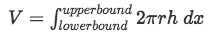Equation 1: Shell Method about y axis pt.12

Notice how the radius of the cylinder depends on x. In addition, the height of the cylinder is between the x-axis and the curve. Hence we can say that: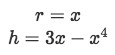Equation 1: Shell Method about y axis pt.13
• Plugging everything in our shell method formula gives us: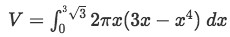Equation 1: Shell Method about y axis pt.14
• Factoring 2π out of the integral and distributing x gives: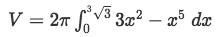Equation 1: Shell Method about y axis pt.15
• Integrating and evaluating gives: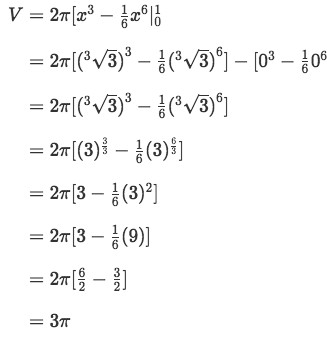Equation 1: Shell Method about y axis pt.16

So this is how shell method integration looks like around the y-axis, but what around the x-axis? Let's do a question with that.

## Shell Method With X Axis Rotation

Question 2: Find the volume of the solid by rotating the positive region bounded by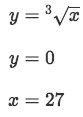Equation 2: Shell Method about x axis pt.1
• First, let's take a look at the region we are bounded by:Graph 5: Shell Method about x axis pt.2
• Note that the formula for the shell method around x-axis is: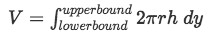Equation 2: Shell Method about x axis pt.3
• Notice the dy here. This means we have to make sure that everything is in terms of y. So changing our curve in terms of y will lead us to have: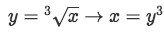Equation 2: Shell Method about x axis pt.4
• Our upper bound and lower bound also have to be in terms of y. Looking at the region we just drew we see that the lower bound is at y=0, and the upper bound is the intersection between x=27 and x=y^3. Solving for the intersection we see that: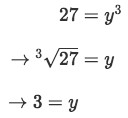Equation 2: Shell Method about x axis pt.5
• So the upper bound is 3. Now what is the radius and height? We need to look at what the graph looks like when it is rotated around the x axis.Graph 6: Shell Method about x axis pt.6
• Notice that the cylindrical shell is now sideways. The radius of the circle depends on y (r=y), and the height of the cylindrical shell is between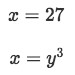Equation 2: Shell Method about x axis pt.7

So the height would be the subtraction of the two. In other words,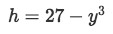Equation 2: Shell Method about x axis pt.8
• Now we have everything to plug into the formula.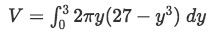Equation 2: Shell Method about x axis pt.9
• Factoring 2π out of the integral and distributing the y we have: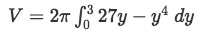Equation 2: Shell Method about x axis pt.10
• Integrating and evaluating we have that: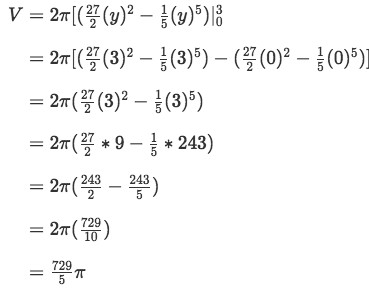Equation 2: Shell Method about x axis pt.11

which is the volume of the solid.

Note that this question can also be solved from using the disk method. Recall the disk method formula for x-axis rotations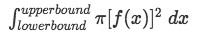Equation 3: Disk method about x axis pt.1
• The bounds are different here because they are in terms of x. However from the graph we see that the lower bound is x=0 and upper bound is x=27. So plugging everything we have: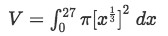Equation 3: Disk method about x axis pt.2
• Factoring π out of the integral and multiplying the exponents we have: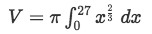Equation 3: Disk method about x axis pt.3
• Integrating gives us: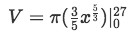Equation 3: Disk method about x axis pt.4
• Evaluating will give us: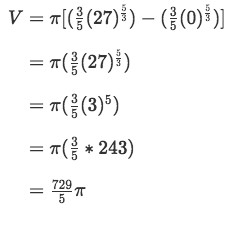Equation 3: Disk method about x axis pt.5

As you can see, we get the same answer. Hence, both shell and disk method works here.

## Shell Method with X-Value Rotation

Let's take a look at a question which involves rotation around a specific x value.

Question 3: Find the volume of the solid obtained by rotating the positive region bounded by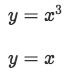Equation 4: Shell Method about x value pt.1

About x=2

• First of all, let's draw the curves and see where the region is.Graph 7: Shell Method about x value pt.2
• So that is the positive region, but what are the bounds in integrating this region? We need to find the intersections of the curves to determine that. Setting the two curves equal to each other we have: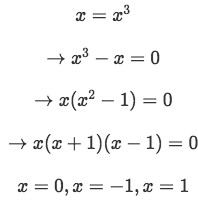Equation 4: Shell Method about x value pt.3

However notice that we are only dealing with positive regions, so we are going to exclude x=-1. Hence, the two x values are x=0, and x=1.

• Note when x=0, then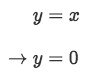Equation 4: Shell Method about x value pt.4

And when x=1, then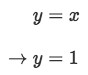Equation 4: Shell Method about x value pt.5
• So we have the points (0,0) and (1,1). Putting these in our graph we will have the following:Graph 8: Shell Method about x value pt.6
• Now we are going to rotate this graph around x=2.Graph 9: Shell Method about x value pt.7

You can see that from the rotation, we can visualize cylindrical shells that are facing upward. We can also see that radius of this cylinder will be difference of 2 and the y=x curve. The height of cylinder will be the difference between the two curves. In other words,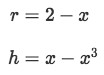Equation 4: Shell Method about x value pt.8
• If you didn't understand why the radius and height are those equations, then you might be able to see it in the next graph.Graph 10: Shell Method about x value pt.9
• Notice how everything is in terms of x. Therefore our lower bounds and upper bounds should be in terms of x as well. So in this case, the lower bound is x=0 and the upper bound is x=1. Recall the shell method formula in terms of x is:Equation 4: Shell Method about x value pt.10
• Plugging everything in we have: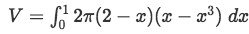Equation 4: Shell Method about x value pt.11
• Factoring 2π out of the integral and distributing we have: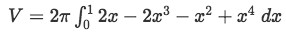Equation 4: Shell Method about x value pt.12
• Integrating and evaluating gives: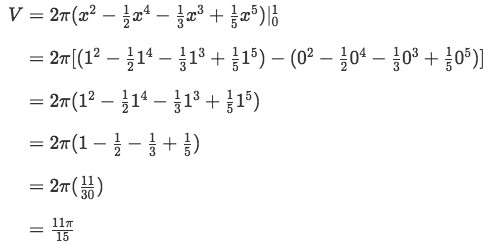Equation 4: Shell Method about x value pt.13

which is the volume of the solid. Keep in mind that this is also solvable with washer's method.

If you want to look at more examples of finding the volume of solid using the shell method, then I suggest you look at this link.

http://tutorial.math.lamar.edu/Classes/CalcI/VolumeWithCylinder.aspx

Paul covers a lot of questions using the shell method

### Volumes of solids of revolution - Shell method

#### Lessons

##### Find the volume of the solid obtained by rotating the positive region bounded by $y=x^3$, $y=x$